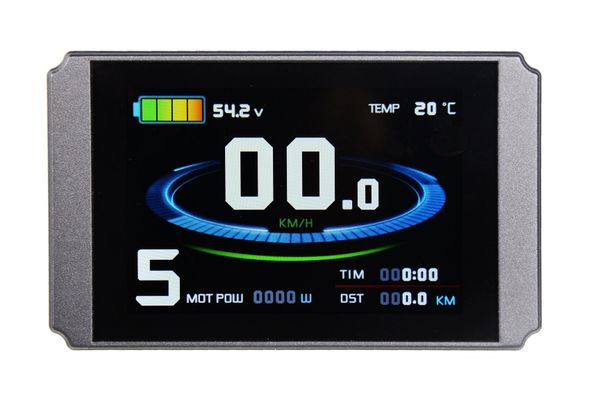\$99.99

Color LCD with USB port for the Rad ebikes. Unlock the speed limiter to 45mph and unleash the potential power of your Rad ebike! This LCD will only work with our 25 & 35a controller (Rad) and can be ordered here https://electrobikeworld.com/products/35a-controller-rad  &

Recommended setting see last photo or

P1 = 100

P2 = 5

P3 = 1

P4 = 0

P5 = 0

C1 = 3

C2 = 0

C3 = 1

C4 = 0

C5 = 2

C6 = 3

C7 = 1

C8 = 0

C9 = 0

C10 = n

C11 = 0

C12 = 4

C13 = 0

C14 = 1

L1=0

L2=0

L3=1

L4=5